MTHH 022 PP Length of The Hypotenuse for A Right Triangle Questions

Nefyna1234

Just \$7 Welcome

Mathematics

MTHH 022

pinecrest prepatory

MTHH

# MTHH 022 PP Length of The Hypotenuse for A Right Triangle

### Question Description

I’m working on a algebra practice test / quiz and need an explanation to help me understand better.

## Questions 1-2

Use this information to answer questions 1 and 2:

Joe is hiking in national park wilderness. His radio transmits and receives signals as far as the horizon. Use the formula:  , where d is the line-of-sight distance to the horizon in miles and h is height in feet.

### Question1.

Joe holds his transmitter at a height of 6 ft. About how far is it from the transmitter to the horizon?

undefined

3 mi

B.

5 mi

C.

7 mi

D.

9 mi

### Question2.

Joe sets his receiver on the ground. What is the farthest away that a transmitter 4 ft above the ground can be in order to reach Joe?

undefined

0 mi

B.

1.2 mi

C.

2.4 mi

D.

6 mi

## Questions 3-38

Select the response that best completes the statement or answers the question.

### Question3.

In a right triangle, find the length of the hypotenuse if the two legs are 36 m and 48 m.

undefined

5 m

B.

2 m

C.

50 m

D.

60 m

### Question4.

What is the length of the third side of a right triangle if one leg is 6 m and the hypotenuse is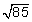m?

undefined

7 m

B.

8 m

C.

9 m

D.

10 m

### Question5.

Using A(0, –2) and B(–6, –9), find the length of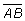rounded to the nearest tenth.

undefined

4.6

B.

9.2

C.

9.3

D.

4.7

### Question6.

Find the midpoint ofif A(8, 11) and B(–5, 2).

undefined

(7, 3)

B.

(9, 13)

C.

(2, 7)

D.

(1.5, 6.5)

### Question7.

What is the length of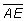in this diagram? (Both triangles are right triangles.)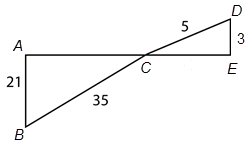undefined

4

B.

32

C.

28

D.

24

### Question8.

Is a triangle with sides, 24ft., 32ft., and 42ft. a right triangle?

undefined

yes

B.

no

### Question9.

What is x, to the nearest tenth, in this 45°-45°-90° triangle?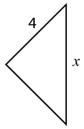undefined

8.7

B.

8

C.

5.7

D.

2

### Question10.

In a 30°-60°-90° triangle, the length of the longer leg is 10. To the nearest tenth, what is the length of the hypotenuse?

undefined

5.0

B.

6.7

C.

11.6

D.

17.3

### Question11.

How high on a wall will a 12-ft ladder reach if the base of the ladder is 3 ft from the wall?

undefined

4.0 ft.

B.

10.0 ft.

C.

11.6 ft.

D.

12 ft.

### Question12.

How high on a wall will a 12-ft ladder reach if the ladder forms a 60° angle with the ground?

undefined

10.4 ft.

B.

8.0 ft.

C.

6.9 ft.

D.

6.0 ft.

### Question13.

If a ramp has an angle of elevation of 19° and a length of 35 ft, what is the height in inches of the ramp at its high end?

undefined

11 in.

B.

12 in.

C.

137 in.

D.

420 in.

### Question14.

A radio transmitting tower casts a shadow that extends 344 feet from the base of the tower. If a line connecting the sun, the top of the tower, and the end of the shadow forms an angle of depression of 16°, approximately how high is the tower?

undefined

344 ft.

B.

99 ft.

C.

1,2008 ft.

D.

1,248 ft.

### Question15.

Which of the following is the best estimate of ?

undefined

6

B.

7

C.

8

D.

9

### Question16.

Which of the following is the best estimate of  ?

undefined

9

B.

10

C.

11

D.

12

### Question17.

Which of the following is a rational number?

undefined

B.

0.93993…

C.

D.

3.474477…

### Question18.

Which of the following is an irrational number?

undefined

743,743

B.

C.

0.2323

D.

0.151155…

### Question19.

Which of the following could be the lengths of the sides of a right triangle?

undefined

2, 6,

B.

5, 12, 13

C.

36, 64, 100

D.

7, 8, 11

### Question20.

Which of the following could be the lengths of the sides of a 30°-60°-90° triangle?

undefined

3, 3,

B.

6, , 23

C.

9, , 18

D.

5,

### Question21.

Find sin 28° to four decimal places.

undefined

0.4695

B.

0.4848

C.

0.8829

D.

0.5317

### Question22.

A right triangle has legs of lengths 7 m and 9 m. Which of the following is the best estimate of the length of the hypotenuse?

undefined

10 m

B.

11 m

C.

12 m

D.

13 m

### Question23.

Simplify this square root:

undefined

–40

B.

8

C.

9

D.

–9

### Question24.

Estimate this square root to the nearest integer:

undefined

5

B.

6

C.

7

D.

8

### Question25.

Identify this number as rational or irrational:

undefined

rational

B.

irrational

### Question26.

In this right triangle, what is the missing length of to the nearest tenth?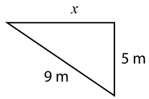undefined

4 m

B.

7.5 m

C.

56 m

D.

8.5 m

### Question27.

What is the distance between these two points, (–8, –9), (1, 2), rounded to the nearest tenth?

undefined

49.2

B.

14.2

C.

7.1

D.

10.9

### Question28.

Find the midpoint between these two points: M(9, –4) and P(2, 8).

undefined

(12, –7)

B.

(6, 5)

C.

(5.5, 2)

D.

(5, 2.5)

### Question29.

Find the missing length of the hypotenuse in this right triangle.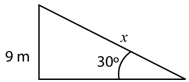undefined

9 m

B.

4.5 m

C.

16 m

D.

18 m

### Question30.

What is the value of tan 29°rounded to four decimal places?

undefined

0.5543

B.

0.4848

C.

0.5540

D.

0.8746

### Question31.

Estimate to the nearest integer:

undefined

3

B.

8

C.

6

D.

7

### Question32.

Simplify the square root:

undefined

B.

C.

2.25

D.

5

### Question33.

A rectangular park measures 300 ft by 400 ft.  A sidewalk runs diagonally from one corner to the opposite corner.  What is the length of the sidewalk?

undefined

700 ft.

B.

50 ft.

C.

1200 ft.

D.

500 ft.

### Question34.

Find the distance (or length of the segment) between the two points: G(4, 7) and H(-2, -3).

undefined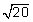B.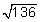C.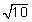D.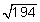### Question35.

Find the midpoint between these two points: G(4, 7) and H(-2, -3).

undefined

(1, 2)

B.

(3, 5)

C.

(6.5, ?2.5)

D.

(?1, ?2)

### Question36.

One leg of a 45°-45°-90° right triangle measures 14 cm.  What is the perimeter?

undefined

56 cm

B.

14 cm

C.

28 + 14 cm

D.

42 cm

### Question37.

A surveyor standing 2,277 ft from the base of a building measured a 31° angle to the topmost point.  To the nearest ft, how tall is the building?

undefined

74 ft.

B.

1952 ft.

C.

3789 ft.

D.

1368 ft.

### Question38.

Find the value of tan 8°.  Round to four decimal places.

undefined

82.8750

B.

0.9923

C.

0.1405

D.

0.1392

### Question39.

Karl is standing 80 ft from the base of a tree.  He sees the top of the tree from an angle of elevation of 42°.  His eye is 4.5 feet off the ground.  How tall is the tree?

undefined

72 ft.

B.

77 ft.

C.

58 ft.

D.

64 ft.

### Question40.

Which of these three lengths will form a right triangle?

undefined

10, 15, 20

B.

3, 4, 6

C.

7, 11, 12

D.

20, 21, 29